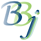# BBjTopLevelWindow::isMinimized

## Description

In BBj 10.0 and higher, this method returns whether the BBjWindow is minimized.

## Syntax

 Return Value Method boolean isMinimized()

None.

## Return Value

Returns whether the BBjWindow is minimized (1=Minimized, 0=Not minimized).

None.

## Example

 REM Determine if a window is minimized REM Obtain the instance of the BBjAPI object LET myAPI!=BBjAPI()   REM Open the SysGui device SYSGUI=UNT OPEN (SYSGUI)"X0"   REM Obtain the instance of the BBjSysGui object LET mySysGui!=myAPI!.getSysGui()   REM Set addWindow param values X=10 Y=10 WIDTH=200 HEIGHT=200   REM Create a window myWindow! = mySysGui!.addWindow(X,Y,WIDTH,HEIGHT,"BBj Window")   REM Minimize the window myWindow!.minimize() REM Determine if the window is minimized MINIMIZED = myWindow!.isMinimized() myWindow!.restore() REM Register the CALLBACK routines CALLBACK(ON_CLOSE,APP_CLOSE,mySysGui!.getContext())   REM Process Events PROCESS_EVENTS   REM Callback routine called when the user closes the application window APP_CLOSE: RELEASE